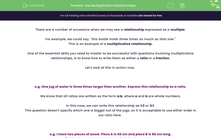# Use Multiplicative Relationships

In this worksheet, students will express multiplicative relationships in terms of ratios and fractions, cancelling them into their simplest by finding the HCF.Key stage:  KS 4

Year:  GCSE

GCSE Subjects:   Maths

GCSE Boards:   AQA, Eduqas, Pearson Edexcel, OCR,

Difficulty level:#### Worksheet Overview

There are a number of occasions when we may see a relationship expressed as a multiple.

For example, we could say, "this bottle holds three times as much as that one."

This is an example of a multiplicative relationship.

One of the essential skills you need to master to be successful with questions involving multiplicative relationships, is to know how to write them as either a ratio or a fraction.

Let's look at this in action now.

e.g. One jug of water is three times larger than another. Express this relationship as a ratio.

We know that all ratios are written as the form a:b, where a and b are whole numbers.

In this case, we can write this relationship as 1:3 or 3:1.

This question doesn't specify which one is bigger out of the jugs, so it is acceptable to use either order in our ratio here.

e.g. I have two pieces of wood. Piece A is 60 cm and piece B is 80 cm long.

a) Write the lengths of A:B as a ratio in its simplest form.

We start by writing each number simply as a ratio, in the order expressed in the question:

60:80

We can then cancel this ratio down into its simplest form by finding the Highest Common Factor (HCF) of both numbers.

Here the HCF is 20:

60 ÷ 20 = 3

80 ÷ 20 = 4

So the simplest possible form of this ratio is 3:4

b) Find the length of B as a fraction of A.

In this question, we are asked to find B as a fraction of A, so B must go on the top of the fraction and A on the bottom, like this:

 80 60

This fraction can then be cancelled down if we divide by a HCF of 20:

 80 ÷ 20 = 4 60 ÷ 20 3

In this activity, we will express multiplicative relationships in terms of ratios and fractions, cancelling them into their simplest by finding the HCF or using them to find missing variables.

### What is EdPlace?

We're your National Curriculum aligned online education content provider helping each child succeed in English, maths and science from year 1 to GCSE. With an EdPlace account you’ll be able to track and measure progress, helping each child achieve their best. We build confidence and attainment by personalising each child’s learning at a level that suits them.

Get started